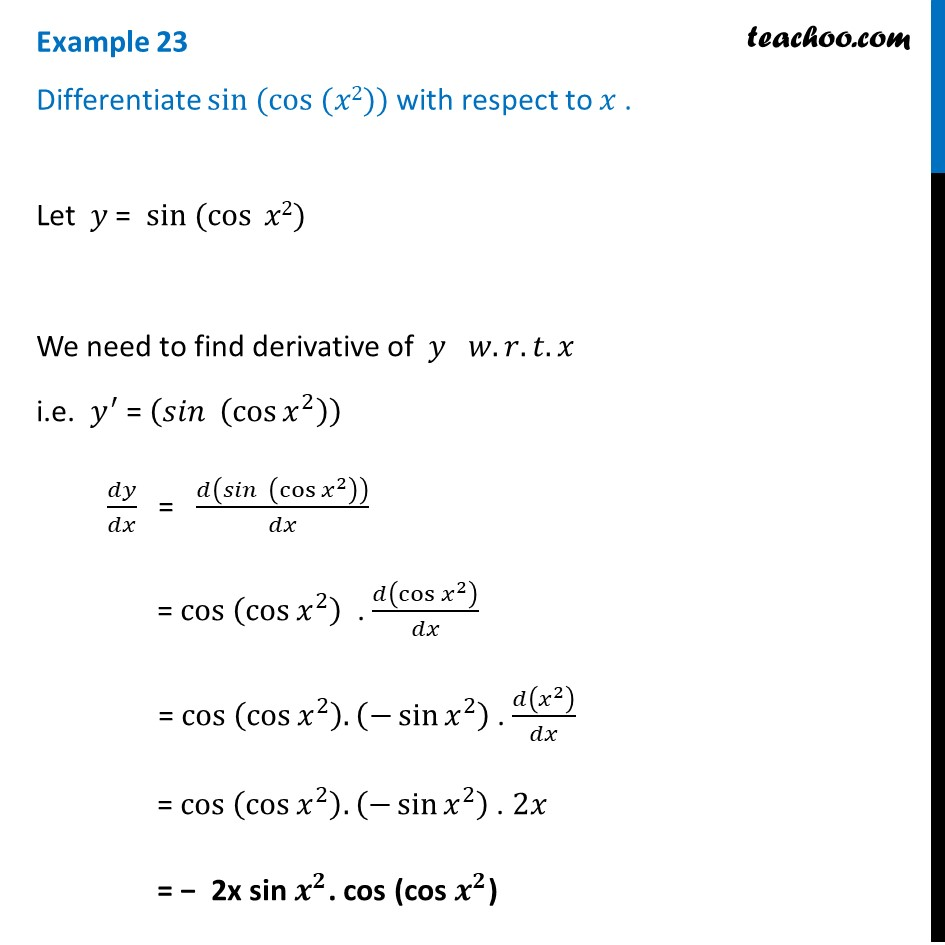Finding derivative of a function by chain rule

Chapter 5 Class 12 Continuity and Differentiability (Term 1)
Concept wise### Transcript

Example 23 Differentiate sin ⁡(cos ⁡(𝑥2)) with respect to 𝑥 .Let 𝑦 = " " sin ⁡(cos ⁡𝑥2) We need to find derivative of 𝑦 𝑤.𝑟.𝑡.𝑥 i.e. 𝑦^′ = (𝑠𝑖𝑛⁡〖 (cos⁡〖𝑥^2 〗 )〗 ) 𝑑𝑦/𝑑𝑥 = 𝑑(𝑠𝑖𝑛⁡〖 (cos⁡〖𝑥^2 〗 )〗 )/𝑑𝑥 = cos (cos⁡〖𝑥^2 〗 ) . 𝑑(cos⁡〖𝑥^2 〗 )/𝑑𝑥 = cos (cos⁡〖𝑥^2 〗 ). (−sin⁡〖𝑥^2 〗 ) . 𝑑(𝑥^2 )/𝑑𝑥 = cos (cos⁡〖𝑥^2 〗 ). (−sin⁡〖𝑥^2 〗 ) . 2𝑥 = − 2x sin 𝒙^𝟐. cos (cos 𝒙^𝟐)